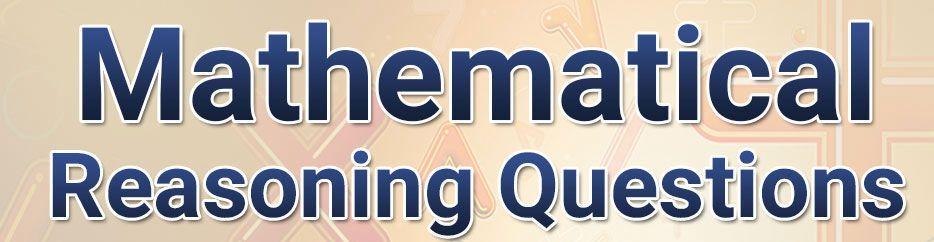# Reasoning Questions With Answers For All Competitive Exams 11-02-2019Reasoning is an important subject which is mostly asked in all the examinations. Bihar forest guard study material Bihar Police Forest Guard Previous Papers with Answers IQ Test, IQ test puzzles, Check IQ for employ, Check IQ for kids, Interview IQ Test, Company IQ Test, MBA IQ Test, CAT Syllogism Puzzles Venn Diagram intelligence Reasoning in Hindi and English means rationality, thought.Daily Science Notes G.K Notes Updates (11-02-2019) for RAS IAS, SSC GD CGL Clerk UPSC Indian History GK 1st Grade, 2nd grade, 3rd grade REET, Teacher, B.Ed, RSMSSB .

### Mathematical Reasoning Questions with Answers(11-02-2019)(Part 2)

Question(1).If P means ×, Q means ÷, R means + & S means –, then what is the value of 154 Q 14 S 7 P 3 R 25?

Daily Visit GK on myshort.in

(A) 35

(B) 57

(C) 42

(D) 15

Question(2).Which interchange of signs will correct the following equation?

35 + 7  ×  5 ÷ 5 – 6 = 24

(A) × & –

(B) + & ×

(C) ÷ & +

(D) – & +

Question(3).Which signs & numbers should be interchanged so the given equation will be correct?

(12÷6) + 3 × 7 = 42

(A) + & ×

(B) 6 & 7

(C) ÷ & +

(D) 12 & 3

Question(4).Which of the following equation will be correct if ÷ & +, 12 & 18 are interchanged with each other?

(A) (90×18) + 18 = 60

(B) (18+6) ÷ 12 = 2

(C) (72÷18) × 18 = 72

(D) (12+6) × 18 = 36

Question(5). Which set of signs should be put at the place of *in the following?

25*2*6=4*11*0

(A) ×, –, ×,+

(B) ÷, –, ×,+

(C) ×,=,+, –

(D) ×, +,+, ×

Question(6).Select the appropriate sequence of mathematical signs, so the given equation will be balanced after interchanging all *s with signs in equation.

9*4*22*14

(A) × = –

(B) × – =

(C) = – ×

(D) – × =

Ans .   (D)

Ans .   (C)

Ans .   (C)

Ans .   (D)

Ans .   (A)

Ans .   (B)

### Questions of Series Completion with  Answers (05-2-2019) Part(1)

Buy online Rajasthan gk book Railway JE CBT REET PAtwari Book SSC CGL Clerk GD Book Buy online Rajasthan gk book Railway JE CBT REET PAtwari Book SSC CGL Clerk GD Book